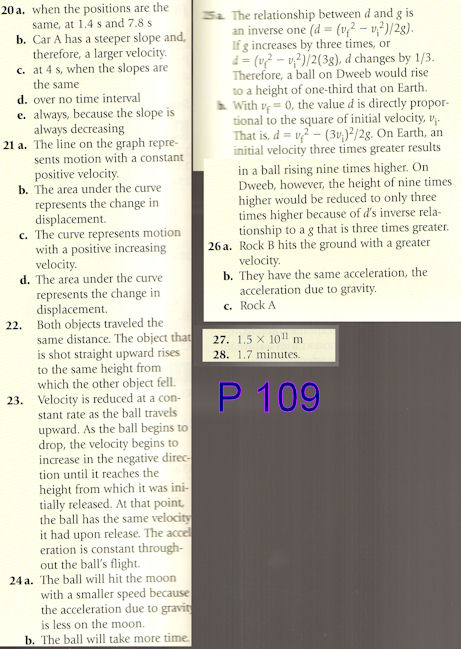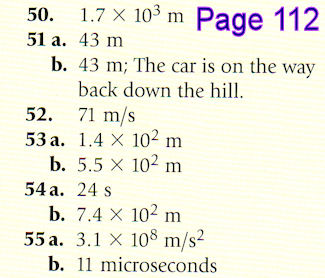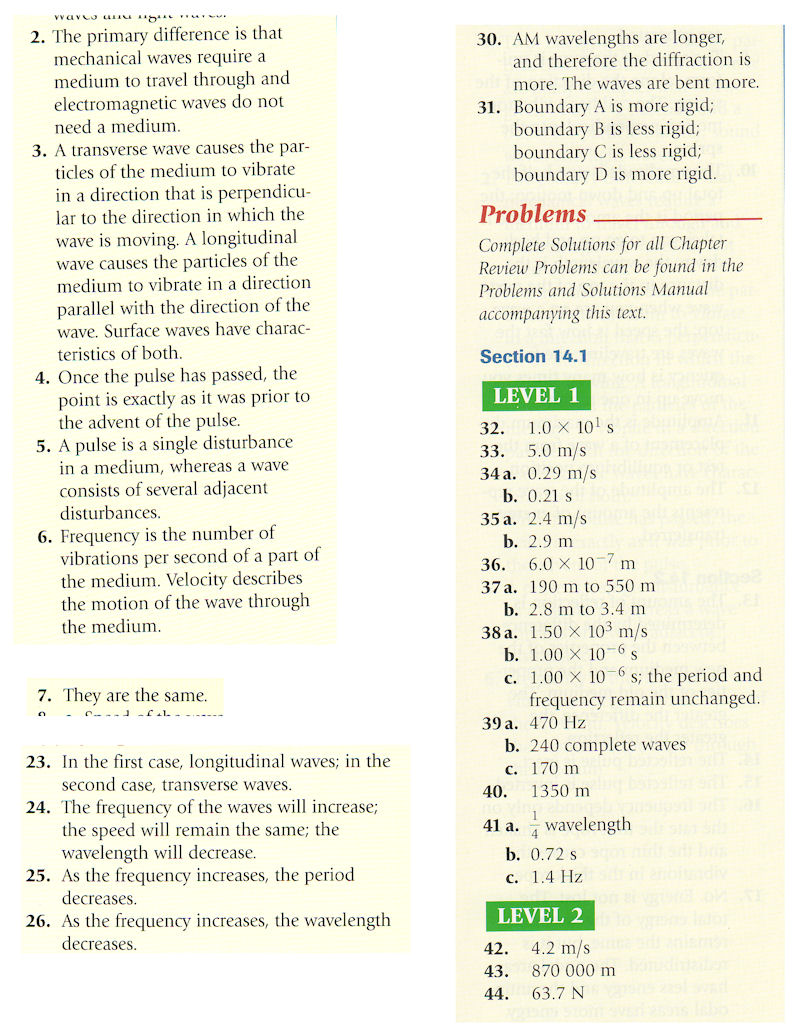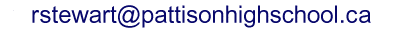## R Stewart, Pattison High School

### Physics 11, Fall 2009

Last update: September 21

P 112

56. 1.6 m thick
57. 2.8 x 102 m/s2 which is 29g
58. a. 247 m/s2 which is 25g
58. b. -207 m/s2 which is 21g
59. We will cover this in class
62. a. Car must travel 5.0 x 102 m. It hits the barrier.
62. b. 22 m/sP. 195

32. 6.1 × 10-9 N

33. 4.17 × 1023 N

34. 5.84 × 10-10 N

37. a. 489 N, b. 4.9 × 102 N

39. a. 6.0 × 1023 kg, b. 5.5 × 103 kg/m3

P. 144

2. A large force is required to accelerate the bicycle and rider. A much smaller force is required to overcome friction and maintain a constant speed.

3. No. If there is no acceleration, the total external force is zero, but there are probably many forces acting on the object. The total is zero, though.

8. The earth does move, but not enough to be measured.

17. A pound (lb) is an English measure of weight equivalent to the weight of 1/2.2 kg on earth's surface. 1 lb = 4.45 N or 1 N = 0.22 lb

19. The equal and opposite forces of Newton's third law act on different objects. The horse applies a force to the cart that is greater than any opposing forces, so the cart accelerates. The fact that the cart also applies a force to the horse is irrelevant in this case.

22. 3.1 × 103 N

23. a. 14.0 m/s,    b. -3.2 × 103 N

24. 126.6 m/s is slower than in problem 21, so the acceleration is not constant. Further, the acceleration in the first half-second was 45 m/s2, not 33 m/s2.

25. 6.3 × 103 N

26. Multiply your mass in (perhaps 65 kg) by 9.8 N/kg (640 N in this case).

27. 2.50 × 102 kg

30. 0.255

31. 5.3 × 104 N

32. 3.0 × 102 N - no

33. a. 1.0 × 101 N
b. 0.20

34. 1.2 m/s2

35. 0.400

36. a. 1.17 m/s2
b. -0.633 m/s2
c. stopping
d. While the elevator is at rest, the scale will read 836.
When it accelerates downward the scale will read less than 836.
The elevator will reach a constant speed, and the scale will read 836.
The elevator will slow, and the scale will read more than 836.
The elevator will stop, and the scale will read 836.

37. a. 4.90 × 102 N
b. 147 N, static friction
c. 49 N, kinetic friction
d. 2.0 × 102 N

38. a. 9.8 m/s2 up
b. 98 m/s up
c. 49 N down
d. 10.0 s after release

39. -2.0 m/s2

P. 218

22. 351 kg·m/s

23. 4.8 kg·m/s

24. 42 m/s

25. a. -1.0 × 102 kg·m/s, b. -5.0 × 102 kg·m/s

26. 20.0 m/s

27. a. 2.13 × 104 kg·m/s, b. 313 N

28. a. 2 × 104 kg·m/s, b. 2 × 104 N, c. The force is exerted by the track through friction.

29. 25 m/s

30. 1.3 × 103 s or 22 minutes

31. a. -2 × 102 kg·m/s, b. -4 × 103 N, c. 4 × 102 kg, d. No, e. There is no way you could hold the kid during an accident

33. a. 1.5 × 102 kg·m/s [down], b. 1.5 × 102 N·s [up], c. 3.0 × 103 N, d. The force is about five times the weight

34. a. Done in class, b. 7.8 × 102 kg·m/s, c. -7.8 × 102 kg·m/s, d. -7.8 × 102 kg·m/s, e. 7.8 × 102 kg·m/s, 6.1 m/s

35. a. ask in class, b. 1.0 × 10-35 kg·m/s, c. 4.0 × 10-4 kg·m/s, d. 1.6 × 10-3 kg·m/s, e. 0.16 m/s

36. 11 m/s

37. 10.6 m/s

38. a. ask in class, b. 5 m/s [W]

39. a. see in class, b. -1.50, c. smaller mass - greater speed, d. forces equal and opposite

40. 0.22 m/s [original direction]

41. a. see in class, b. 0.041 m/s, c. yes - final velocity is positive

42. a. -0.500 kg·m/s, b. -0.995 kg·m/s, c. case b

Page 269 Answers will not be posted tonight.

36.

39.

41.

42.

44.

45.

48.

53.# 46. 0.90 m/s

ASSIGNMENTS

Email: